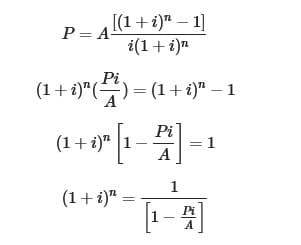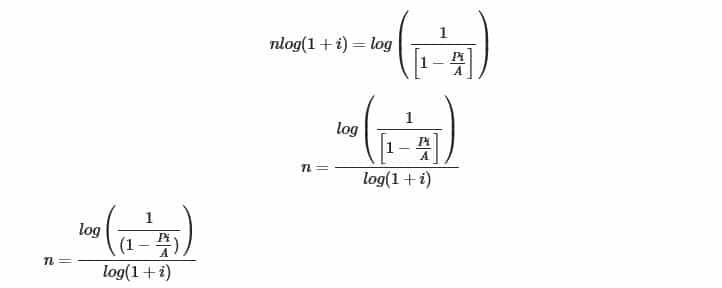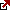# How to calculate number of periods for an annuity ?

Understanding Number of Periods with Present Value (Annuity)
How to solve for n in present value annuity formula? Solving for N is an important factor when completing the equation for the number of periods for an annuity. This equation is a practical solution for finding the total number of periods. A classic example of this equation is solving for the number of payments needed in order to complete a loan. For example, with this equation, one can determine how many payments it will take them to pay back a loan such as a mortgage or auto. If periodic payments are made on a consistent basis, the number of periods will help to determine the end date of payment. It is also important to note that there are many factors that contribute to loans and this equation helps to solve it on an academic level.

If we take Present Value and Annuity formula where “i” is the rate than :If one was offered a loan of \$19,600 with a 1% rate per month, it would take them 22 payment periods at \$1,000 per payment to finish the loan. This is a great method for helping to find the total number of periods needed to successfully pay back the loan. The rate and payments should match when considering this formula. There are many ways to implement this formula when acquiring a loan. This is a great method for helping to determine if a loan is sustainable and can be paid back easily by the borrower. The term and rate should match on a consistent “per period” basis.

It is also important to consider if the loan has an annual rate. For example, if payments are made on a consistent annual basis, an effective annual rate is utilized. The equation for N can be reduced. The equation helps to figure out the consistent term of the loan. Solving for N can be simple and straightforward when using the correct equation. The last payment in a loan term subject to changes. For example, the last payment on a loan can be more or less than the period payment total. The main reason for this is due to fees and extra added costs. For example, when one is granted a loan, there are processing fees and other types of costs added to the final total. These fees are often paid in the last installment of the loan period payment.

The Number of Periods of Annuity from Present Value

A perfect example of this equation would be an auto or home loan. When determining the number of payment periods, you must first determine a few important pieces of information. The loan amount must be known, as well as the number of period payments. If period payments are higher than average, the loan will be paid back quicker. If period payments are lower than average, the loan will take longer to fully complete. By understanding this basic principle, finding the number of payments is easier as all the necessary factors have been covered. There is also an alternative method for solving N. For example, an annuity table can be used with present value. The “middle section” of the table can be used to find the value of N as well.

Latest posts by Fxigor (see all)

Fxigor

Igor has been a trader since 2007. Currently, Igor works for several prop trading companies. He is an expert in financial niche, long-term trading, and weekly technical levels. The primary field of Igor's research is the application of machine learning in algorithmic trading. Education: Computer Engineering and Ph.D. in machine learning. Igor regularly publishes trading-related videos on the Fxigor Youtube channel. To contact Igor write on: igor@forex.in.rs

Trade gold and silver. Visit the broker's page and start trading high liquidity spot metals - the most traded instruments in the world.Diversify your savings with a gold IRA.

Gold & silver portfolio-building assistance from product professionals.

If you want to trade stocks try: